# Posts tagged as “binary search”

Given an array of integers that is already sorted in ascending order, find two numbers such that they add up to a specific target number.

The function twoSum should return indices of the two numbers such that they add up to the target, where index1 must be less than index2. Please note that your returned answers (both index1 and index2) are not zero-based.

You may assume that each input would have exactly one solution and you may not use the same element twice.

Input: numbers={2, 7, 11, 15}, target=9
Output: index1=1, index2=2

Solution:

C++ / two pointers

Time complexity: O(n)

Space complexity: O(1)

C++ / Binary search

Related Problems:

Problem:

Given a sorted array consisting of only integers where every element appears twice except for one element which appears once. Find this single element that appears only once.

Example 1:

Example 2:

Note: Your solution should run in O(log n) time and O(1) space.

Idea:

Binary search.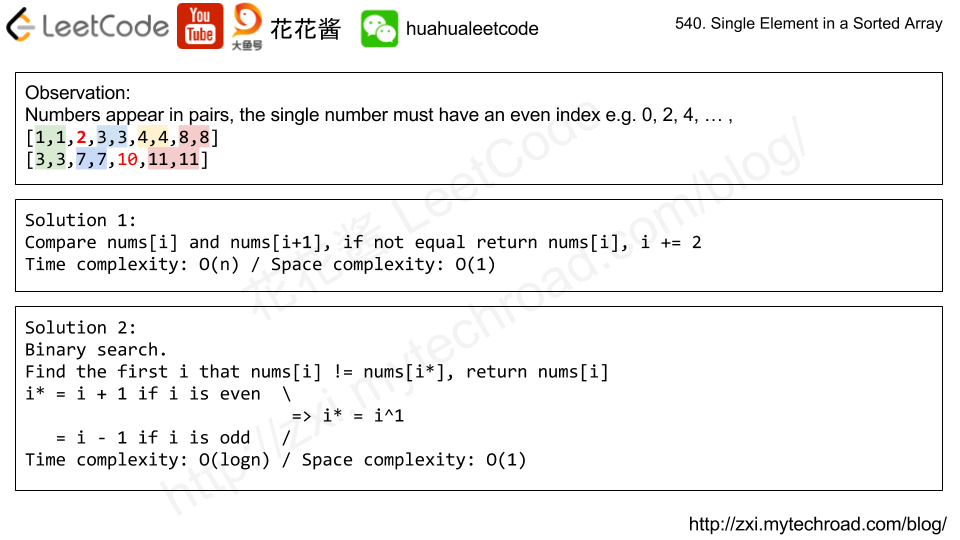Time complexity: O(logn)

Space complexity: O(1)

Solution:

C++

Problem:

Given a list of sorted characters letters containing only lowercase letters, and given a target letter target, find the smallest element in the list that is larger than the given target.

Letters also wrap around. For example, if the target is target = 'z' and letters = ['a', 'b'], the answer is 'a'.

Examples:

Note:

1. letters has a length in range [2, 10000].
2. letters consists of lowercase letters, and contains at least 2 unique letters.
3. target is a lowercase letter.

Idea:

1. Scan the array Time complexity: O(n)
2. Binary search Time complexity: O(logn)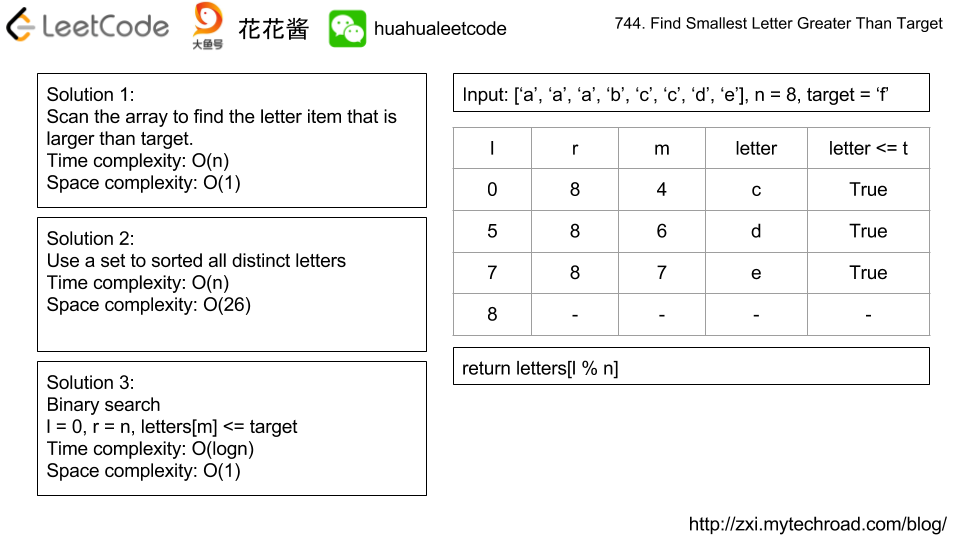Solution 1:

C++ / Scan

C++ / Set

C++ / Binary Search

Problem:

There are two sorted arrays nums1 and nums2 of size m and n respectively.

Find the median of the two sorted arrays. The overall run time complexity should be O(log (m+n)).

Example 1:

Example 2:

Idea:

Binary Search

Time complexity: O(log(min(n1,n2)))

Space complexity: O(1)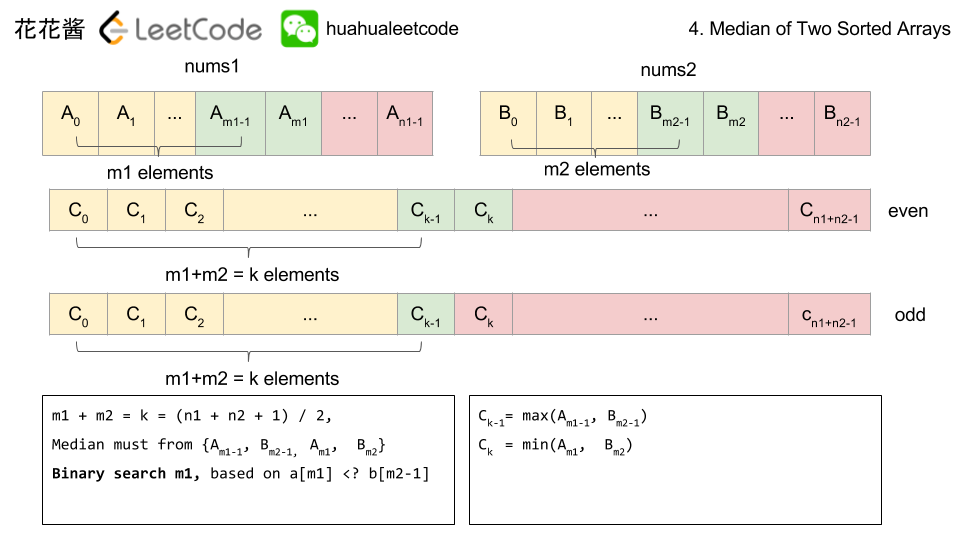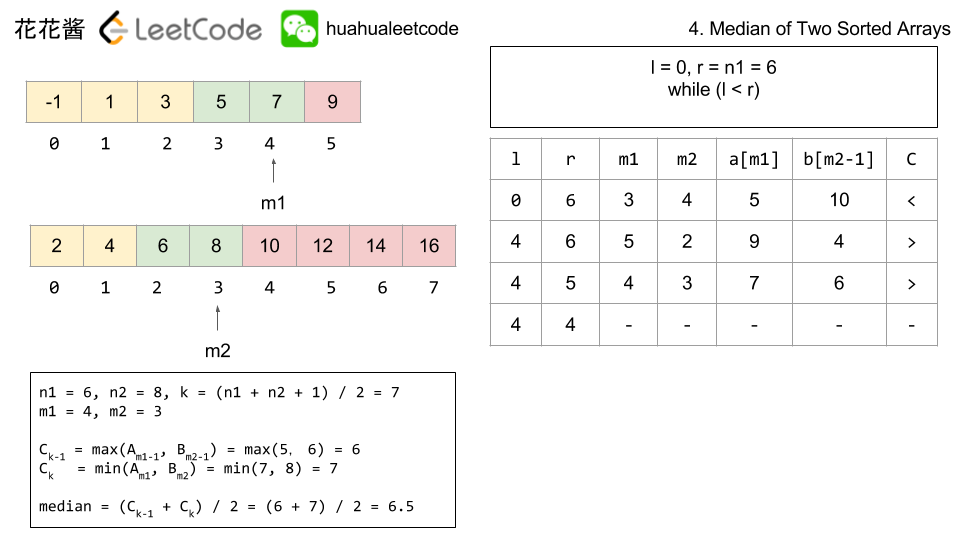# Related Problem:

Problem:

Given an integer array, return the k-th smallest distance among all the pairs. The distance of a pair (A, B) is defined as the absolute difference between A and B.

Example 1:

Note:

1. 2 <= len(nums) <= 10000.
2. 0 <= nums[i] < 1000000.
3. 1 <= k <= len(nums) * (len(nums) - 1) / 2.

Idea

Bucket sort

Binary search / dp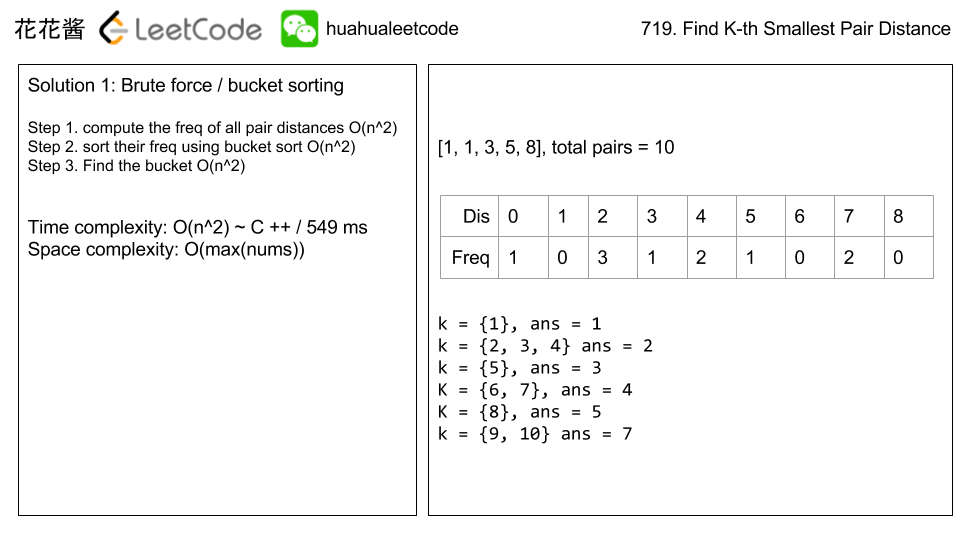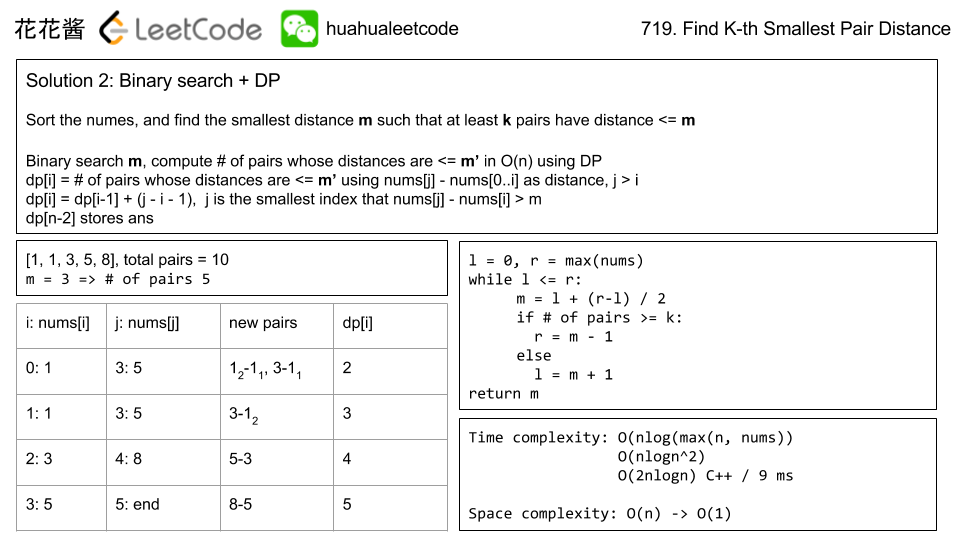Solution

C++ / binary search

C++ / bucket sort w/ vector O(n^2)

Related Problems:

Mission News Theme by Compete Themes.